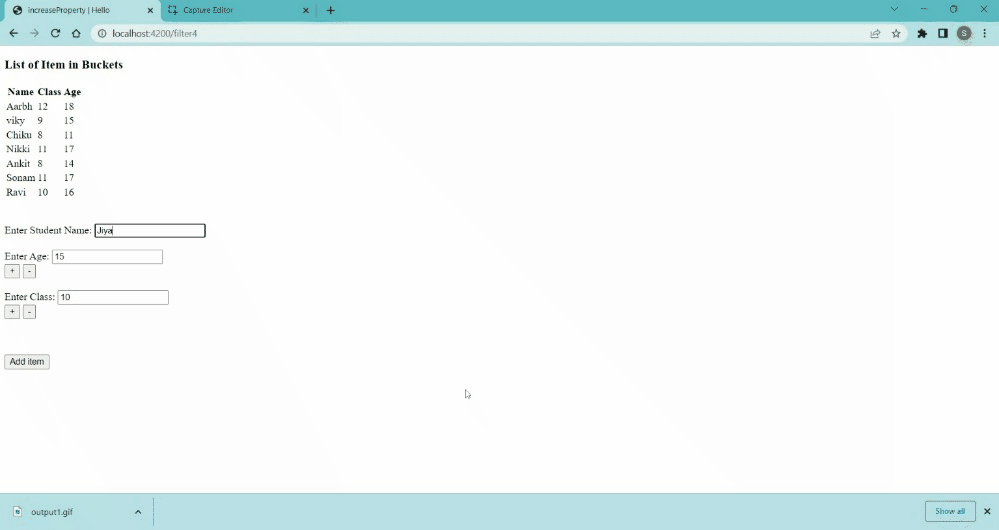Open in App
Not now

# Ember.js Observable decrementProperty() Method

• Last Updated : 26 Dec, 2022

Ember.js is an open-source JavaScript framework used for developing large client-side web applications which are based on Model-View-Controller (MVC) architecture. Ember.js is one of the most widely used front-end application frameworks. It is made to speed up development and increase productivity. Currently, it is utilized by a large number of websites, including Square, Discourse, Groupon, Linked In, Live Nation, Twitch, and Chipotle.

The decrementProperty() method is used to decrease the value of property to some amount.

Syntax:

`decrementProperty(keyName,decrement);`

Parameters:

• keyName: It is the name of the property whose value we want to decrement.
• decrement: It is the value to which we want to decrement. The default value is 1.

Return Value: This method returns a new property value.

Steps to install and run Ember.js:

Step 1: To run the following examples you will need to have an ember project with you. To create one, you will need to install ember-cli first. Write the below code in the terminal:

`npm install ember-cli`

Step 2: Now you can create the project by typing in the following piece of code:

`ember new <project-name> --lang en`

To start the server, type:

`ember serve`

Example 1: Type the following code to generate the route for this example:

`ember generate route decrement1`

app/routes/decrement1.js

## Javascript

 `import Route from ``'@ember/routing/route'``;` `import EmberObject from ``'@ember/object'``;`   `export ``default` `class WebsitesRoute extends Route {`   `    ``temp;` `    ``model() {` `        ``return` `"Increment Property"``;` `    ``}` `    ``setupController(controller, model) {` `        ``super``.setupController(controller, model);` `        ``// controller.set('food', this.food);` `        ``controller.set(``'temp'``, ``this``.temp);` `        ``controller.set(``'temp2'``, ``this``.temp2);` `    ``}` `}`

app/controllers/decrement1.js

## Javascript

 `import Ember from ``'ember'``;` `import EmberObject from ``'@ember/object'``;`   `const Student = EmberObject.extend({` `    ``toStringExtension() {` `        ``return` `this``.get(``'food'``);` `    ``}` `});` `export ``default` `Ember.Controller.extend({` `    ``class: 10,` `    ``age: 15,` `    ``student: [` `        ``Student.create({` `            ``Name: ``'Aarbh'``,` `            ``class: 12,` `            ``age: 18,` `        ``}),` `        ``Student.create({` `            ``Name: ``'viky'``,` `            ``class: 9,` `            ``age: 15,` `        ``}),` `        ``Student.create({` `            ``Name: ``'Chiku'``,` `            ``class: 8,` `            ``age: 11,` `        ``}),` `        ``Student.create({` `            ``Name: ``'Nikki'``,` `            ``class: 11,` `            ``age: 17,` `        ``}),` `        ``Student.create({` `            ``Name: ``'Ankit'``,` `            ``class: 8,` `            ``age: 14,` `        ``}),` `        ``Student.create({` `            ``Name: ``'Sonam'``,` `            ``class: 11,` `            ``age: 17,` `        ``}),` `        ``Student.create({` `            ``Name: ``'Ravi'``,` `            ``class: 10,` `            ``age: 16,` `        ``}),` `    ``],` `    ``actions: {` `        ``older(data) {` `            ``this``.incrementProperty(data);` `        ``},` `        ``younger(data) {` `            ``this``.decrementProperty(data);` `        ``},`   `        ``addItem(data, data1, data2) {`   `            ``let temp = Student.create({` `                ``Name: data,` `                ``class: data1,` `                ``age: data2` `            ``});` `            ``alert(temp.toString() + ``' Student Added in list'``);` `            ``this``.student.addObject(temp);` `        ``}` `    ``}` `});`

app/templates/decrement1.hbs

## HTML

 `{{page-title "decrementProperty"}}` `<``h3``>List of Item in Buckets` `<``table``>` `    ``<``tr``>` `        ``<``th``> Name ` `        ``<``th``>Class ` `        ``<``th``>Age ` `      ``` `      ``{{#each this.student as |website|}}` `        ``<``tr``>` `             ``<``td``>{{website.Name}}` `              ``<``td``>{{website.class}}` `              ``<``td``>{{website.age}}` `        ``` `      ``{{/each}}` `` `<``br` `/><``br` `/>` `<``div``>` `    ``<``label``>Enter Student Name: ` `      ``{{input value=this.temp2}}` `` `<``br` `/>` `<``div``>` `    ``<``label``>Enter Age: ` `      ``{{input value=this.age}}` `` `<``input` `    ``type``=``"button"` `id``=``"increase"` `    ``value``=``"+"` `{{action "older" "age"}} />` `<``input` `    ``type``=``"button"` `id``=``"decrease"` `    ``value``=``"-"` `{{action "younger" "age"}} />` `<``br` `/>` `<``br` `/>` `<``div``>` `    ``<``label``>Enter Class: ` `      ``{{input value=this.class}}` `` `<``input` `type``=``"button"` `id``=``"increase"` `    ``value``=``"+"` `{{action "older" "class"}} />` `<``input` `type``=``"button"` `id``=``"decrease"` `    ``value``=``"-"` `{{action "younger" "class"}} />` `<``br` `/>` `<``br` `/>` `<``br` `/><``br` `/>` `<``input` `    ``type``=``"button"` `      ``id``=``"all-students"` `      ``value``=``"Add item"` `      ``{{action "addItem" this.temp2 this.class this.age}}` `/>` `{{outlet}}`

Output:decrementProperty output1

My Personal Notes arrow_drop_up
Related Articles